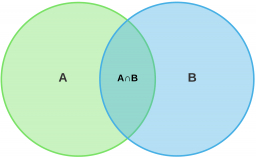# A math

A math teacher teaches Geometry and Precalculus. 37% of students take both math classes, and only 39% take just Geometry. What is the probability that a student will attend Precalculus given that they attended Geometry? What is the percentage of students that participated in Precalculus?

P =  24 %
p2 =  94.8718 %

### Step-by-step explanation:Did you find an error or inaccuracy? Feel free to write us. Thank you!

Tips for related online calculators
Need help calculating sum, simplifying, or multiplying fractions? Try our fraction calculator.